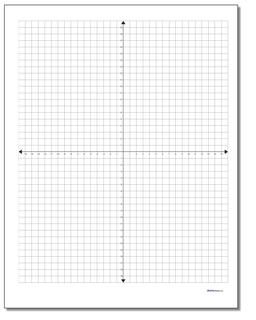# Math Worksheets: Coordinate Plane: Coordinate Plane: Cartesian Metric Graph Paper (Second Worksheet)## Cartesian Metric Graph Paper (Second Template)

PropertyValue
DescriptionCartesian Metric Graph Paper: Printable coordinate planes in inch and metric dimensions in various sizes, great for plotting equations, geometry problems or other similar math problems. These are full four-quadrant graphs. These coordinate planes have labels directly along the x axis and y axis. (Second Template)
Resource TypeTemplate